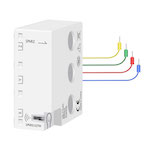# # TuYa SPM02

ModelSPM02
VendorTuYa
DescriptionSmart energy monitor for 3P+N system
Exposesvoltage_X, voltage_Y, voltage_Z, power_X, power_Y, power_Z, current_X, current_Y, current_Z, energy, produced_energy, linkquality
Picture## # Options

How to use device type specific configuration

• `energy_precision`: Number of digits after decimal point for energy, takes into effect on next report of device. The value must be a number with a minimum value of `0` and with a with a maximum value of `3`

• `energy_calibration`: Calibrates the energy value (percentual offset), takes into effect on next report of device. The value must be a number.

## # Exposes

### # Voltage X (numeric)

Measured electrical potential value (phase X). Value can be found in the published state on the `voltage_X` property. It's not possible to read (`/get`) or write (`/set`) this value. The unit of this value is `V`.

### # Voltage Y (numeric)

Measured electrical potential value (phase Y). Value can be found in the published state on the `voltage_Y` property. It's not possible to read (`/get`) or write (`/set`) this value. The unit of this value is `V`.

### # Voltage Z (numeric)

Measured electrical potential value (phase Z). Value can be found in the published state on the `voltage_Z` property. It's not possible to read (`/get`) or write (`/set`) this value. The unit of this value is `V`.

### # Power X (numeric)

Instantaneous measured power (phase X). Value can be found in the published state on the `power_X` property. It's not possible to read (`/get`) or write (`/set`) this value. The unit of this value is `W`.

### # Power Y (numeric)

Instantaneous measured power (phase Y). Value can be found in the published state on the `power_Y` property. It's not possible to read (`/get`) or write (`/set`) this value. The unit of this value is `W`.

### # Power Z (numeric)

Instantaneous measured power (phase Z). Value can be found in the published state on the `power_Z` property. It's not possible to read (`/get`) or write (`/set`) this value. The unit of this value is `W`.

### # Current X (numeric)

Instantaneous measured electrical current (phase X). Value can be found in the published state on the `current_X` property. It's not possible to read (`/get`) or write (`/set`) this value. The unit of this value is `A`.

### # Current Y (numeric)

Instantaneous measured electrical current (phase Y). Value can be found in the published state on the `current_Y` property. It's not possible to read (`/get`) or write (`/set`) this value. The unit of this value is `A`.

### # Current Z (numeric)

Instantaneous measured electrical current (phase Z). Value can be found in the published state on the `current_Z` property. It's not possible to read (`/get`) or write (`/set`) this value. The unit of this value is `A`.

### # Energy (numeric)

Total forward active energy. Value can be found in the published state on the `energy` property. It's not possible to read (`/get`) or write (`/set`) this value. The unit of this value is `kWh`.

### # Produced energy (numeric)

Total reverse active energy. Value can be found in the published state on the `produced_energy` property. It's not possible to read (`/get`) or write (`/set`) this value. The unit of this value is `kWh`.

Link quality (signal strength). Value can be found in the published state on the `linkquality` property. It's not possible to read (`/get`) or write (`/set`) this value. The minimal value is `0` and the maximum value is `255`. The unit of this value is `lqi`.